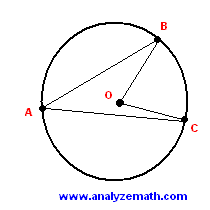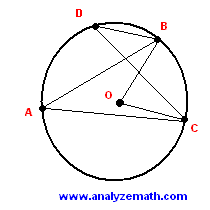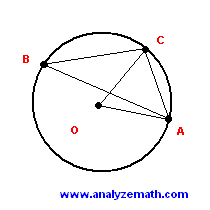# Inscribed and Central Angles in Circles

Definitions and theorems related to inscribed and central angles in circles are discussed using examples. See also interactive tutorial on central and inscribed angles.

## Definitions

1 - A central angle of a circle is an angle whose vertex is located at the center of the circle. Angle BOC in the figure below.
2 - An inscribed angle is an angle whose vertex is on a circle and whose sides each intersect the circle at another point. Angle CAB in the figure below.## Theorem

1 - An inscribed angle is half the measure of the central angle intercepting the same arc.
angle BAC = (1 / 2) angle BOC

angle BDC = (1 / 2) angle BOC

2 - Two or more inscribed angles intercepting the same arc are equal.
angle BAC = angle BDC### Problem

In the figure below chord CA has a length of 12 cm. The circle of center O has a radius of 14 cm. Find an approximate value (2 decimal places) to the size of the inscribed angle CBA.Solution to Problem 1

• We first calculate the central angle COA. Triangle COA is an isosceles triangle since length of CO = length of AO = radius = 14 cm. We use the cosine law to find cos (angle COA).
CA 2 = CO 2 + AO 2 - 2 CO AO cos (angle COA)
• Substitute CA, CO and AO by their numerical values and express cos(angle COA) as follows
cos(angle COA) = [ 14 2 + 14 2 - 12 2 ] / [2 * 14 * 14 ]
= 31 / 49
• Size of angle COA is given by.
Angle COA = arcos(31 / 49)
• According to the theorem above, the size of angle CBA is equal to half the size of angle COA.
Angle CBA = (1 / 2) arcos(31 / 49)
= 25.38 degrees (approximated to 2 decimal places)

## More References and Links to Geometry

Geometry Tutorials, Problems and Interactive Applets.